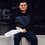# Calling All Cauch Polyatatoes

Hello all! I was recently reading a proof of the AM-GM inequality by George Polya in my book titled "The Cauchy-Schwarz Master Class" by professor J. Michael Steele. I think it's an extraordinary, beautiful proof, but there's one step that I don't quite understand. I'll post a relatively quick proof, pointing out my area of confusion when I get there.

So, we want to prove that for a sequence of reals $a_k$ with $1\leq k\leq n$ and a sequence of reals $p_k$ with $1\leq k \leq n$ such that $\sum_{k=1}^{n} p_k = 1$, that

$a_1^{p_1}a_2^{p_2}a_3^{p_3}...a_n^{p_n}\leq a_1p_1+a_2p_2+a_3p_3+...+a_np_n$

Polya's proof begins with the observation that $1+x\leq e^x$ which can easily be seen graphically with equality occurring only at $x=0$. If we make the change of variables $x\mapsto x-1$, our initial observation becomes $x\leq e^{x-1}$. Applying this to our sequence $a_k$ we get $a_k\leq e^{a_k -1}$

$a_k^{p_k}\leq e^{a_kp_k - p_k}$ now, we can see that $G=\prod_{k=1}^{n} a_k^{p_k} \leq \exp \left(\sum_{k=1}^{n} a_kp_k - \sum_{k=1}^{n} p_k\right)$ $G=\prod_{k=1}^{n} a_k^{p_k} \leq \exp \left(\sum_{k=1}^{n} a_kp_k - 1\right)$ however, we can also see from one of our initial observation that $A=\sum_{k=1}^{n} a_kp_k \leq \exp \left(\sum_{k=1}^{n} a_kp_k -1\right)$ which is the same bound we just found for $G$. So we could say

$A, G \leq \exp \left (\sum_{k=1}^{n} a_kp_k -1 \right )$ So we have related $A$ and $G$ by inequality, but we have not separated them. Now we look closer at the case where $A$ and $G$ are equal to the expression on the right. The idea of "normalization" then comes to mind. Normalization was discussed earlier in the book, but $\color{limegreen}{\text{this is precisely where I got lost.}}$ What Steele does is the following:

Define a new sequence $\alpha_k$ with $1\leq k \leq n$ where we have $\alpha_k = \frac{a_k}{A}$ where we have $A=a_1p_1+a_2p_2+a_3p_3+...+a_np_n$

Applying our earlier bound for $G$ for our new variables $\alpha_k$, we get $\prod_{k=1}^{n} \alpha_k^{p_k} \leq \exp \left(\sum_{k=1}^{n} \alpha_kp_k-1\right)$ $\prod_{k=1}^{n} \left(\frac{a_k}{A}\right)^{p_k} \leq \exp \left(\sum_{k=1}^{n} \frac{a_k}{A}p_k-1\right)$ Now, Steele implies that $\sum_{k=1}^{n} \frac{a_k}{A}p_k = 1$ and it follows that $\exp \left(\sum_{k=1}^{n} \frac{a_k}{A}p_k-1\right) = 1$

Now from here, showing that the AM-GM inequality holds is easy. The only place that brings me confusion is the purpose of introducing and defining the sequence $\alpha_k$ in the way that Steele does. If someone could help me out with this, it would be greatly appreciated! Consider this a recommendation for "The Cauchy-Schwarz Master Class," it's a fantastic book and you'd all love it!Note by Ryan Tamburrino
6 years, 6 months ago

This discussion board is a place to discuss our Daily Challenges and the math and science related to those challenges. Explanations are more than just a solution — they should explain the steps and thinking strategies that you used to obtain the solution. Comments should further the discussion of math and science.

When posting on Brilliant:

• Use the emojis to react to an explanation, whether you're congratulating a job well done , or just really confused .
• Ask specific questions about the challenge or the steps in somebody's explanation. Well-posed questions can add a lot to the discussion, but posting "I don't understand!" doesn't help anyone.
• Try to contribute something new to the discussion, whether it is an extension, generalization or other idea related to the challenge.

MarkdownAppears as
*italics* or _italics_ italics
**bold** or __bold__ bold
- bulleted- list
• bulleted
• list
1. numbered2. list
1. numbered
2. list
Note: you must add a full line of space before and after lists for them to show up correctly
paragraph 1paragraph 2

paragraph 1

paragraph 2

[example link](https://brilliant.org)example link
> This is a quote
This is a quote
    # I indented these lines
# 4 spaces, and now they show
# up as a code block.

print "hello world"
# I indented these lines
# 4 spaces, and now they show
# up as a code block.

print "hello world"
MathAppears as
Remember to wrap math in $$ ... $$ or $ ... $ to ensure proper formatting.
2 \times 3 $2 \times 3$
2^{34} $2^{34}$
a_{i-1} $a_{i-1}$
\frac{2}{3} $\frac{2}{3}$
\sqrt{2} $\sqrt{2}$
\sum_{i=1}^3 $\sum_{i=1}^3$
\sin \theta $\sin \theta$
\boxed{123} $\boxed{123}$

Sort by:

Update: a few minutes later, while proofreading the post for errors, I looked at what I was confused with and I have seen the light! I now understand why the sequence was introduced.

$\sum_{k=1}^{n} \frac{a_k}{A}p_k = \frac{a_1p_1+a_2p_2+a_3p_3+...+a_np_n}{A} = \frac{A}{A} = 1$

Seems so obvious now, but I guess that's how it always goes. I will leave the post and proof up because I think it's a great proof for the more general version of the AM-GM Inequality. Also, I feel like this is a prime example of how sometimes, one just needs to take a step back and look at problems from a fresh perspective.

- 6 years, 6 months ago

A bit of a confusing point for me too, but fantastic proof nonetheless.

- 6 years, 6 months ago

This is great! Can you add this to the AM-GM wiki? Thanks!

Staff - 6 years, 6 months ago

Sure, I'd love to!

- 6 years, 6 months ago